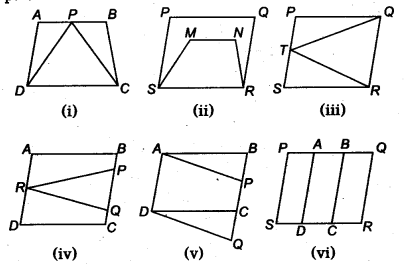NCERT Solutions for Class 9 Maths Chapter 10 Areas of Parallelograms and Triangles Ex 10.1 are part of NCERT Solutions for Class 9 Maths. Here we have given NCERT Solutions for Class 9 Maths Chapter 10 Areas of Parallelograms and Triangles Ex 10.1.

 Board CBSE Textbook NCERT Class Class 9 Subject Maths Chapter Chapter 10 Chapter Name Areas of Parallelograms and Triangles Exercise Ex 10.1 Number of Questions Solved 1 Category NCERT Solutions

## NCERT Solutions for Class 9 Maths Chapter 10 Areas of Parallelograms and Triangles Ex 10.1

Question 1.
Which of the following figures lie on the same base and between the same parallels. In such a case, write the common base and the two parallels.Solution:
In Fig. (i), APDC and trape∠ium ABCD Wes on the same base DC and between the same parallel lines AB and DC.
In Fig. (iii), ATRO and parallelogram PQRS lies on the same base RQ and between the same parallel lies RQ and SP.
In Fig. (v), quadrilateral APCD and quadrilateral ABQD lies on the same base AD and between the same parallel lines AD and BQ.

We hope the NCERT Solutions for Class 9 Maths Chapter 10 Areas of Parallelograms and Triangles Ex 10.1 help you. If you have any query regarding NCERT Solutions for Class 9 Maths Chapter 10 Areas of Parallelograms and Triangles Ex 10.1, drop a comment below and we will get back to you at the earliest.